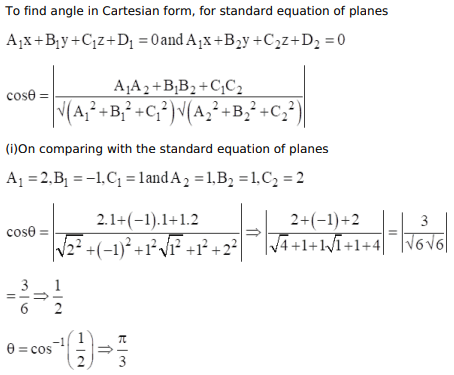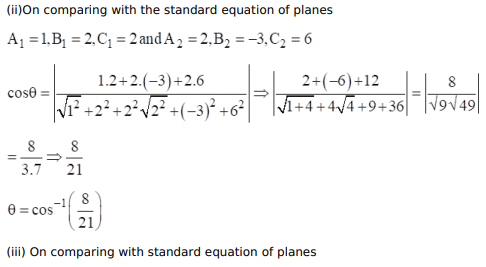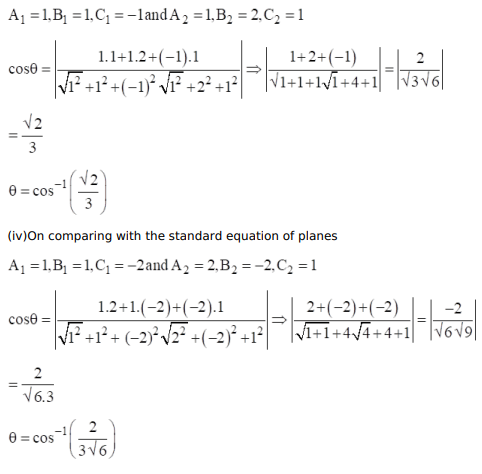# Find the acute angle between the following planes:

Question:

Find the acute angle between the following planes:

(i) $2 x-y+z=5$ and $x+y+2 z=7$

(ii) $x+2 y+2 z=3$ and $2 x-3 y+6 z=8$

(iii) $x+y-z=4$ and $x+2 y+z=9$

(iv) $x+y-2 z=6$ and $2 x-2 y+z=11$

Solution: